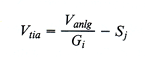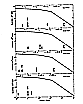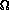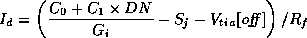VI.A. Processing - Removal of Telescope Transfer Function

# IRAS Explanatory Supplement VI. Flux Reconstruction and Calibration A. Processing - Removal of Telescope Transfer Function

Chapter Contents | Introduction | Authors | References

The electronic chain by which the current caused by photons incident onto the detector is transferred to the signal received at the ground station is described in Section II.C.5. Briefly, the detector acted as a current source as a result of the incident photons. This current was input to a trans-impedance amplifier which transformed the signal into a voltage equaling the total current times the feedback resistor plus a small offset voltage due primarily to the imbalance in the cold JFETs at the inputs to the operational amplifiers. The output voltage from the trans-impedance amplifier was amplified and shaped by analog electronics, fast rise time nuclear particle spikes were removed and the analog voltage was converted into a digital signal for transmission in a compressed format to the Earth. In the processing, the route was reversed. As described below, the received signal, in data numbers, was retraced to the current at the detector.

## A.1 Digital Electronics

Pre-launch ground tests determined that there were no significant errors or non-linearities introduced by the digital electronics or by the analog to digital conversion. Any errors introduced by the compression of digital data on board the telescope and reconstruction at the processing center were assumed to be random and to be in the least significant bit and were ignored. The raw input was therefore assumed to correspond exactly to the output of the analog to digital converter. Since a linear analog to digital converter was assumed, the voltage into the analog to digital converter, i.e., the voltage at the output of the analog chain was given by:

Vanlg = C0 + C1 × DN

where

 Vanlg = voltage at output of the analog amplifier = voltage at the analog digital converter C0 = inherent voltage offset of the analog digital converter DN = observed signal in data numbers. C1 = data number voltage conversion factor (volts/DN)

Preflight measurements showed that any adverse effect on the data due to the multiplexer should be negligible and it was therefore assumed to be linear.

## A.2 Analog Electronics Amplifiers

The analog electronics contained the commandable gains and offsets. The input voltage to this part of the electronics can be recovered by(VI.A.2)

where,

 Vtia = voltage at trans-impedance amplifier output Gi = commandable gain, i = 1, 2, 3. Sj = commandable offset, j = 1, 2,..., 8 .

The effects on the flux calibration due to the pole zero amplifier and the pulse circumvention circuit (deglitcher), which also lie in the analog electronics board between the output of the preamplifier electronics and the commandable offset electronics, were ignored. The analog electronics also contained a filter to remove high frequencies in the signal. Adverse effects due to the filter were considered to be small at the frequencies of interest and were therefore ignored.

## A.3 Trans-impedance Amplifier

In order to recover the extended component of the incident flux, it was necessary to determine, and remove, any electronic baseline offset. The voltage at the output of the trans-impedance amplifier was taken to be of the form:

Vtia[observed] = Id × Rf + Vtia[off]

where Id is the current through the detector and Rf is the feedback resistor.

After launch, the electronic baselines at 60 and 100 µm were found to be affected by the bias boosts induced during crossings of the South Atlantic Anomaly (SAA). The effect is thought to be due to the \ differential heating of the JFETs because of the large current passing through the JFET connected to the detector. This effect was modeled by adding a time dependent exponential function to Vtia[off]

Corr = (a + b × T) × exp(-/)

(V.A.4)

where:

 T = duration of the most recent bias boost a,b = constants= time interval between time of interest and end of most recent bias boost= characteristic decay time of the baseline shift

This correction was added to the electronic baseline value before removing the electronic baseline from the data. For a typical passage through the SAA, the magnitude of this baseline shift was about 2O% and 8O% of the signal from the TFPR at 60 µm and 100 µm. The time constantwas typically 52 minutes at 60 µm and 42 minutes at 100 µm.

## A.4 Removal of Coherent Detector Noise

Detector 5 (100 µm) exhibited a stable, coherent 0.25 Hz waveform. By adding successive 4-second intervals of data coherently, it was possible to determine the shape of the waveform to better than l% of the remaining rms noise. This wave form was then subtracted from the detector 5 data stream. This resulted in an order of magnitude improvement in the rms noise and greatly reduced the number of spurious point source detections for detector 5. After removal of the wave form, the character of the resulting detector 5 data stream was typical of 100 µm detectors.

Detector 19 (25 µm) exhibited a spike, of randomly varying amplitude, every second at sample #9 of the 16 Hz sampled data stream. The typical amplitude was approximately 6-8 times the cleaned rms noise for detector 19. The amplitude was measured by subtracting the average of the neighboring samples, which showed no evidence of electronic cross-talk from the value of the spike sample. If the spike amplitude was less than a maximum allowable amplitude (approximately 10 times the rms noise of the cleaned detector 19 data), then the spiked sample was replaced by the average of its neighboring samples. If the spike amplitude was greater than the maximum allowable amplitude, then the maximum allowable amplitude was subtracted from the spike sample. The latter case can occur in the 6% of detector 19 detections for which the spike appears on top of a point source. If the signal to noise ratio of such a point source after the spike was removed is less than 20, the result will be a "topped" source. This process can lower the correlation coefficient below threshold for some of the weaker sources. Sources brighter than signal to noise ratio ~ 2O will have progressively higher correlation coefficients. After removing the spikes, the overall rms noise for detector 19 was reduced by a factor ~3.

Detector 43 exhibited a 1 Hz waveform that consisted of a positive spike with signal to noise ratio ~2 at sample #9 of the 16 Hz sampled output, followed by a small negative spike, with signal to noise ratio ~½, at sample #10. The amplitude varied randomly, but remained small, with a signal to noise ratio < 3. In this case a constant waveform of the above shape was subtracted, resulting in a reduction of the detector 43 rms noise by a factor of ~1.3. All subtractions were effectively done at the output of the trans-impedance amplifier.

## A.5. Feedback ResistorFigure VI.A.1 The fit used to represent the feedback resistor is shown in the four wavelength bands. The flux densities corresponding to the appropriate voltages across the resistor are shown. The same fit, that shown in Fig. II.C.11, was used for all the detectors. larger largest

The feedback resistors had a nominal resistance of 2 × 1010, but in fact the resistance of the feedback resistor was dependent on the voltage across the resistor. The fit to the feedback resistor used in the data reduction is a three-piece curve shown in Fig. VI.A.1a-d. It is the same fit as shown in Fig. II.C.11. This was the only nonlinearity assumed in the processing.

## A.6 Summary

The processing to remove the instrumental transfer functions can be summarized by the following equation for the current in the detector.(V.A.5)

Chapter Contents | Introduction | Authors | References